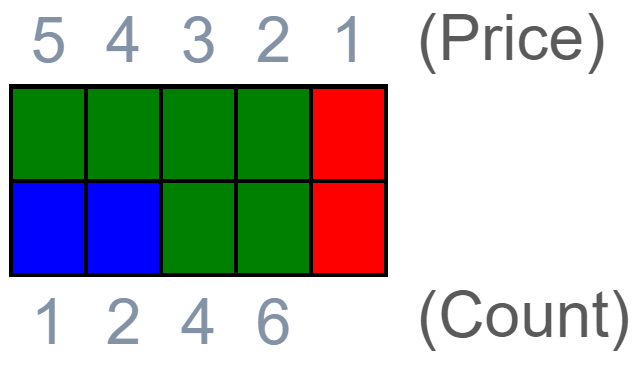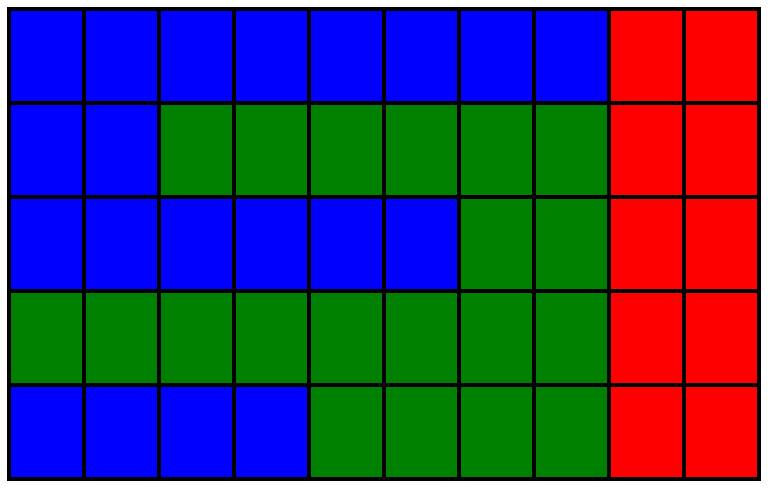# Find The Highest Profit

A Company has several suppliers for its products. For each of the products, the stock is represented by a list of a number of items for each supplier. As items are purchased, the supplier raises the price by `1` per item purchased. Let's assume Amazon's profit on any single item is the same as the number of items the supplier has left.

For example, if a supplier has `4 items`, Amazon's profit on the first item sold is `4`, then `3`, then `2` and the profit of the last one is `1`.

Given a list where each value in the list is the number of the item at a given supplier and also given the number of items to be ordered, write an algorithm to find the highest profit that can be generated for the given product.

Notes: `-10` is smaller than `-1`. If multiple people have the smallest negative balance, return the list in alphabetical order. If nobody has a negative balance, return the list consisting of the string "Nobody has a negative balance".

Write an algorithm to find who in the group has the smallest negative balance.

### Input

The input consists of three arguments:

`numSuppliers`: an `integer` representing the number of suppliers

`inventory`: a `list of long integers` representing the value of the item at a given supplier

`order`: a `long integer` representing the number of items to be ordered.

### Output

Return a `long integer` representing the highest profit that can be generated for the given product.

### Constraints

`1 <= numSuppliers <= 10^5`

`1 <= inventory[i] <= 10 ^ 5`

`0 <= i < numSuppliers`

`1 <= orders <= sum of inventory`

### Examples

#### Example 1:

##### Input:

numSuppliers = `2`

inventory = `[3,5]`

order = `6`

##### Explanation:

There are two suppliers, one with inventory `3` and the other with inventory `5`, and `6` items were ordered The maximum profit is made by selling `1` for `5`, `1` for `4`, and `2` at `3` and `2` at `2` units profit. The two suppliers are left with a unit of product each. The maximum profit generated is `5 + 4 + 2*3 + 2*2 = 19`.Maximizing profit: Green represents units purchased by the marketer, Red squares are products retained by the suppliers. Blue squares are empty.

#### Example 2:

##### Input:

numSuppliers = `5`

inventory = `[2, 8, 4, 10, 6]`

order = `20`

##### Explanation:

There are 5 sellers with inventory = `[2, 8, 4, 10, 6]` and Items ordered are `20`. The marketer will purchase items from any supplier until they have only `2` units left.Green represents units purchased by the marketer, Red squares are products retained by the suppliers. Blue squares are empty.

The maximum profit generated is `10 + 9 + 2*8 + 2*7 + 3*6 + 3*5+ 4*4 + 4*3 = 10 + 9 + 16 + 14 + 18 + 15 + 16 + 12 = 110`.